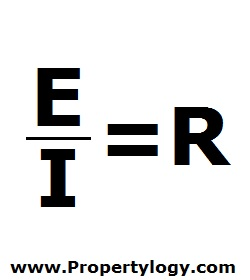Formula - Expense Ratio | Propertylogy
Don't Miss

Formula – Expense Ratio

By on June 8, 2018E/I = R

E – Operating expense

I – Gross income from rental

R – Expense ratio

Sometimes also called the operating ratio, the expense ratio reveals the relationship between operating expenses and rental income.

This can be very useful when comparing properties to invest in.

Expressed as a percentage, it is important to note that mortgage interest expense is not taken into account when determining E because interest expense can vary.

Send this to a friend